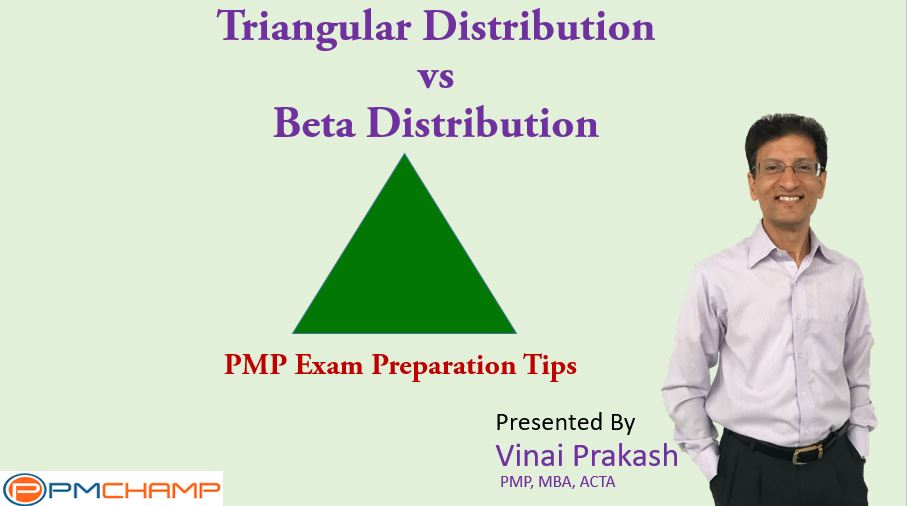# 3 Point Estimate: Triangular Distribution vs Beta Distribution (PERT)PMP aspirants often come across the 3 Point Estimate when studying for the PMP exam for the first time. There are 2 different formulas for the 3 Point Estimate, and it is generally not clear to most project managers as to which one to use, and when, or why.

I have written about the 3 point estimation technique quite some time back, about the 2 different formulas and how to use them. This article takes it further,  to explain some of the basic differences between the two , and make it easy for you to prepare for the PMP exam, as well as apply this useful estimation technique in your projects.

The Three Point Estimate technique is used to arrive at a better estimate of the time required to complete a particular activity, work package, and can be rolled up to the entire project. Further, this technique can be used for Time as well as Cost also.

Triangular Distribution: (P + O + ML ) / 3

This is like a simple average of the three estimates. When plotted in a chart, it usually results in a sharp peak, thus the name Triangular Distribution.

Beta Distribution (PERT): (P + O + 4ML ) / 6

This is a weighted average. More weight is given to the most likely. If plotted against a chart, this beta distribution will result in an more uniform, bell shaped curve, called a normal distribution. This has been tested in several different industries, across different countries, and over different time periods, but has always turned out to be more accurate than  the 3 point estimate. So this method has become the most used and popular method of choice among project managers.When do you use PERT and when do you use Triangular Distribution?

However, the Beta Distribution is preferred in cases where we have a lot of historical data, and is more useful for similar type of projects, and experts are providing this based on historical evidence & experience . If we are doing a project for the first time, and there is no previous history of similar projects to fall back on, it may make sense to use the Triangular distribution, as there is no basis to give more weight to a particular estimate. Thus, a Triangular Distribution is more suited for judgemental data estimates. These are most likely not based on a lot of experience or expertise… but are more of a guess-timates.

As with all estimates, the more accurate your estimates, the better the Triangular and Beta Distribution curves, and the better we are able to estimate the project risk and its completion on time and on cost.

For PMP exam, look for subtle clues in the exam question. If they talk about a new project, no experience, or not much reliable data available, then it is best to use Triangular distribution.

And if the team is made of experts, this is a similar to previous projects, we need a more accurate estimate etc. then it is best to go for the Beta Distribution using the PERT formula of weighted average.

Hope this helps in your PMP exam preparation and in your real life projects. Do drop a note in the comment below…

Cheers,
Vinai Prakash

### 1 thought on “3 Point Estimate: Triangular Distribution vs Beta Distribution (PERT)”

1.I respectfully disagree concerning the accuracy of the PERT estimate vs the Triangular estimate. The PERT “expected value” or mean is usually closer to the most likely value based on the 4x weighting of the most likely value. In my opinion this leads to an understated (optimistic) mean that in practice is almost always LESS than p50. Even the simple mean of the triangular distribution tends to be slightly optimistic usually driven by the asymmetrical (skewed) shape of the distribution. Most estimates using the three point technique set the “worst case” further from the most likely than the best case so you end up with a 10, 20, 40 triangle or something similar. In this example, the PERT EV would be 21.6 and the simple mean 23.3.

In this case the “Most Likely” value has a probability of 33% The 50% probability comes in around 27.8. so the PERT is more optimistic but both PERT and the simple average are both off the mark. Why? The skewed nature of the distribution.

The skewed value is an admission that more can go wrong than can go right. The long tail o the right side of the distribution almost screams “lognormal” which I contend would be a better estimator in most cases than the simple triangular distribution or the PERT Beta.